Function Repository Resource:

# GracefulGraphFromPermutation

Show the graceful graph corresponding to a given permutation

Contributed by: Ed Pegg Jr
 ResourceFunction["GracefulGraphFromPermutation"][perm] shows the graceful graph for the permutation perm.

## Details and Options

A graceful graph has n edges labeled 1 to n, with each edge label equal to the absolute difference between the labels of its vertices.

## Examples

### Basic Examples (1)

Every permutation can be converted to a graceful graph:

 In:=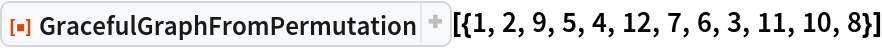Out=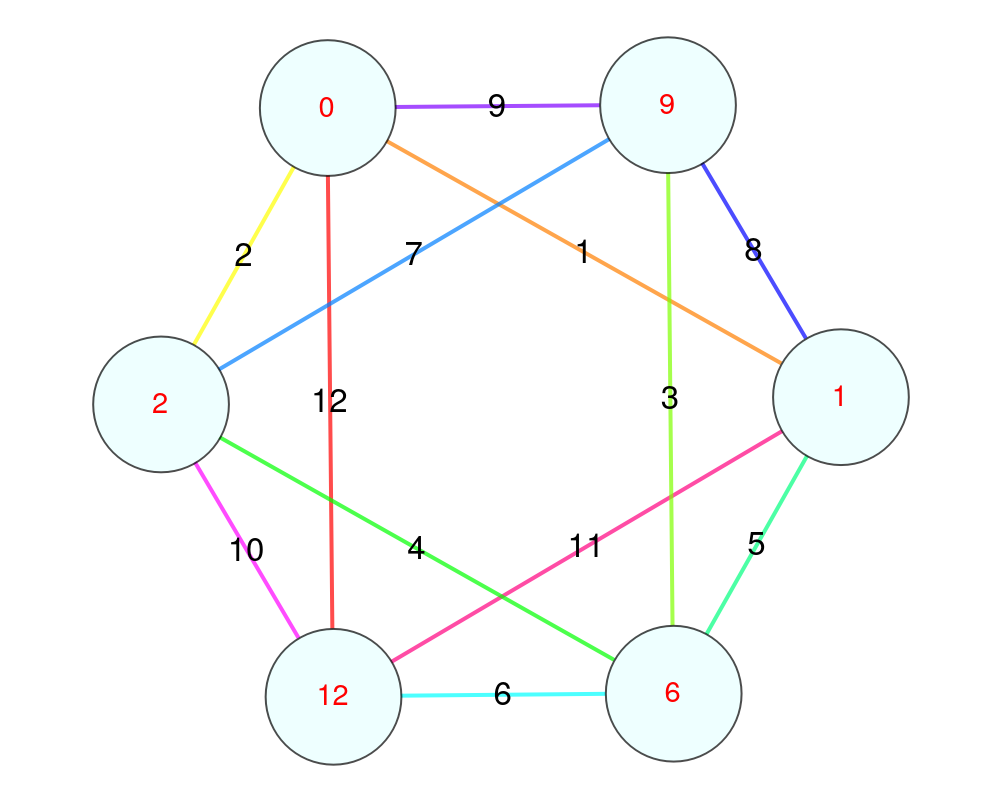### Scope (3)

Some graceful graphs are planar:

 In:=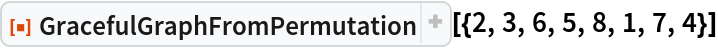Out=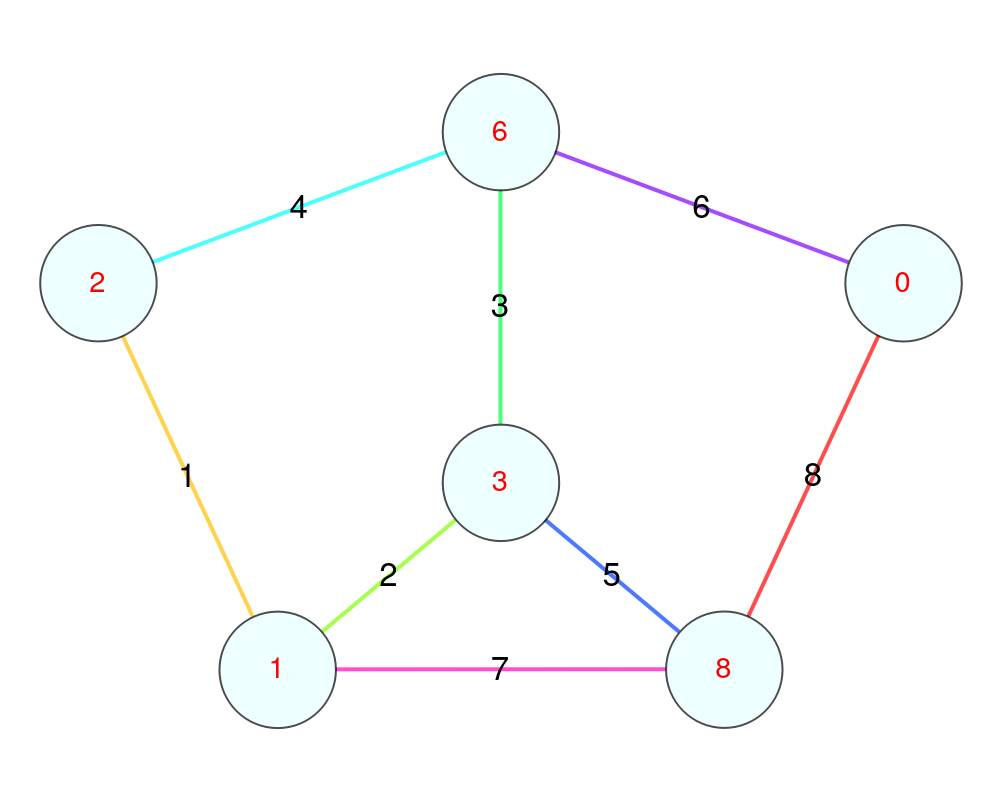Any random permutation can give a graceful graph:

 In:=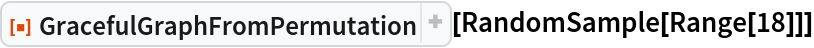Out=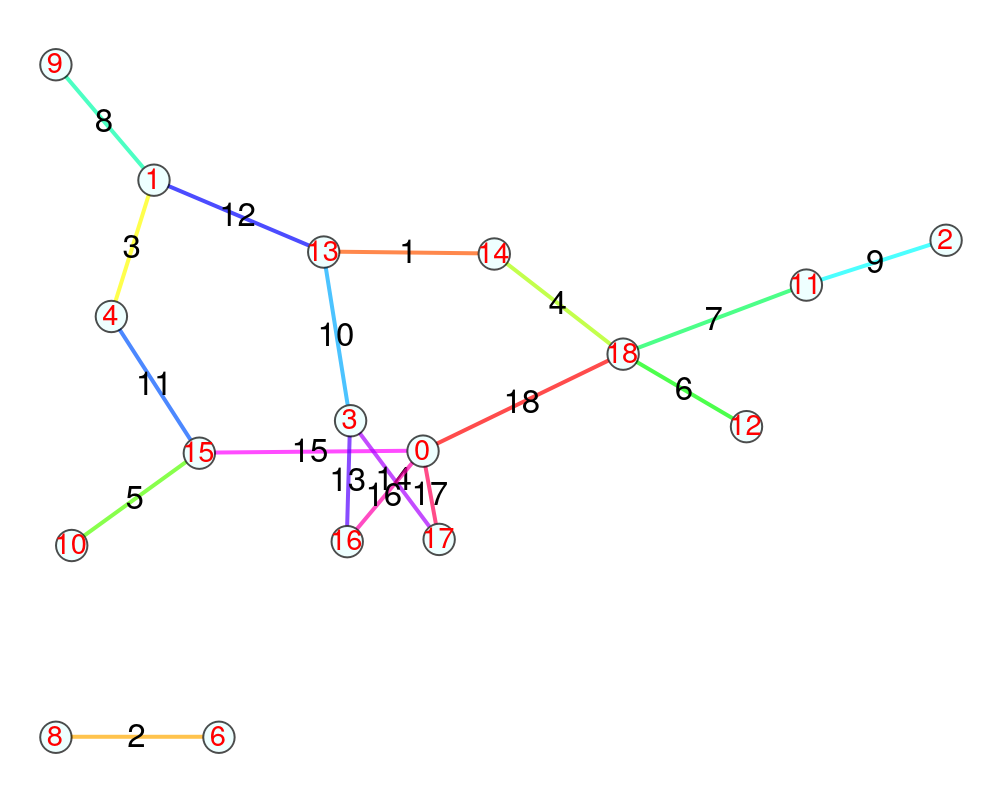Some graceful graphs are trees:

 In:=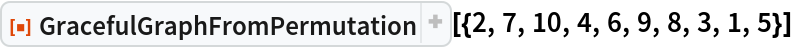Out=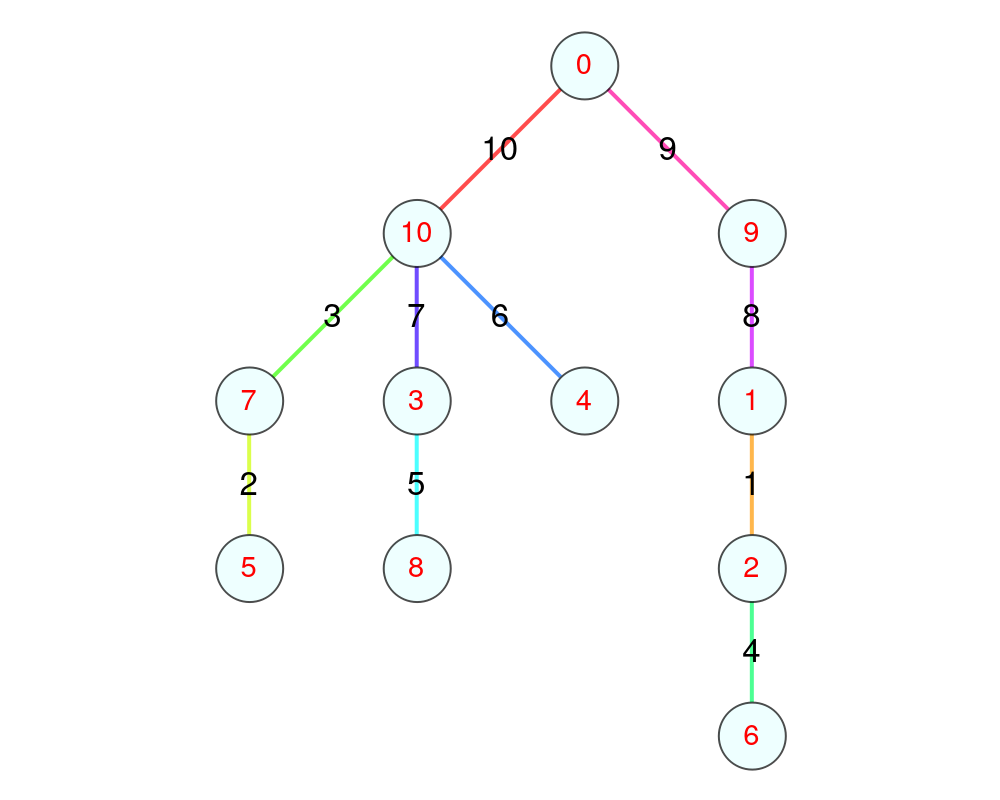## Version History

• 2.0.0 – 15 July 2019
• 1.0.0 – 06 February 2019